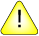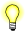This version of this document is no longer maintained. For the latest documentation, see http://www.qnx.com/developers/docs.

# modf(), modff()

Break a number into integral and fractional parts

## Synopsis:

```#include <math.h>

double modf( double value,
double* iptr );

float modff( float value,
float* iptr );```

## Arguments:

value
The value that you want to break into parts.
iptr
A pointer to a location where the function can store the integral part of the number.

## Library:

libm

Use the -l m option to qcc to link against this library.

## Description:

The modf() and modff() functions break the given value into integral and fractional parts, each of which has the same sign as the argument. They store the integral part as a double in the object pointed to by iptr.

## Returns:

The signed fractional part of value.If an error occurs, these functions return 0, but this is also a valid mathematical result. If you want to check for errors, set errno to 0, call the function, and then check errno again. These functions don't change errno if no errors occurred.

## Examples:

```#include <stdio.h>
#include <stdlib.h>
#include <math.h>

int main( void )
{
double integral_value, fractional_part;

fractional_part = modf( 4.5, &integral_value );
printf( "%f %f\n", fractional_part, integral_value );

fractional_part = modf( -4.5, &integral_value );
printf( "%f %f\n", fractional_part, integral_value );

return EXIT_SUCCESS;
}```

produces the output:

```0.500000 4.000000
-0.500000 -4.000000```

## Classification:

Safety:
Cancellation point No
Interrupt handler No
Signal handler No